Static and Kinetic FrictionPage 1

WATCH ALL SLIDES

Slide 1Static and Kinetic Friction

Nerik Yakubov Robert Magee David Chen

Slide 2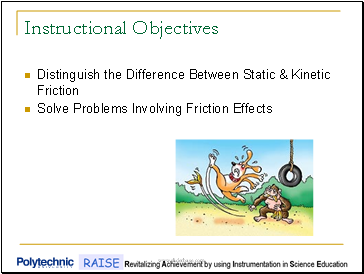Instructional Objectives

Distinguish the Difference Between Static & Kinetic Friction

Solve Problems Involving Friction Effects

Slide 3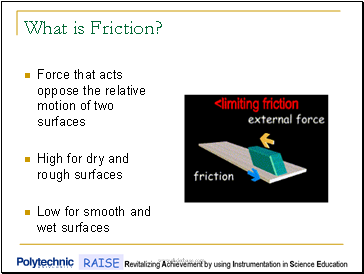What is Friction?

Force that acts oppose the relative motion of two surfaces

High for dry and rough surfaces

Low for smooth and wet surfaces

Slide 4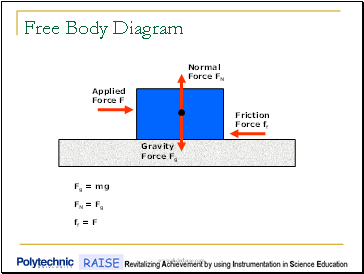Free Body Diagram

Normal Force FN

Friction Force ff

Applied Force F

Gravity Force Fg

Fg = mg

FN = Fg

ff = F

Slide 5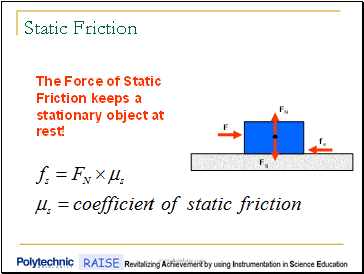Static Friction

FN

fs

F

Fg

The Force of Static Friction keeps a stationary object at rest!

Slide 6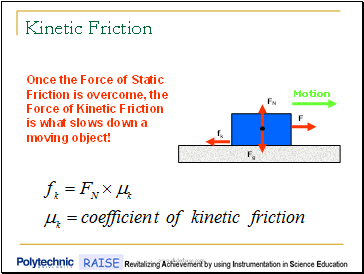Kinetic Friction

FN

fk

F

Fg

Once the Force of Static Friction is overcome, the Force of Kinetic Friction is what slows down a moving object!

Motion

Slide 7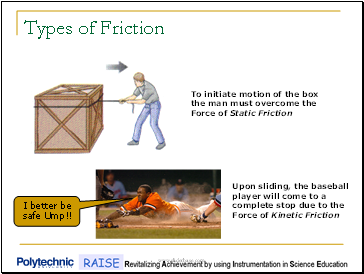Types of Friction

I better be safe Ump!!

To initiate motion of the box the man must overcome the Force of Static Friction

Upon sliding, the baseball player will come to a complete stop due to the Force of Kinetic Friction

Slide 8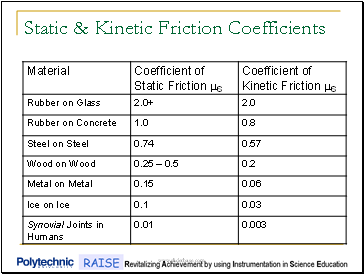Static & Kinetic Friction Coefficients

Slide 9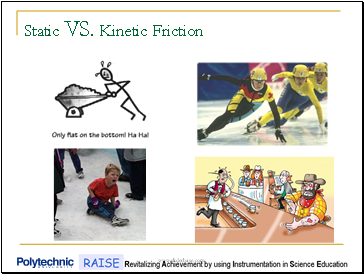Static VS. Kinetic Friction

Slide 10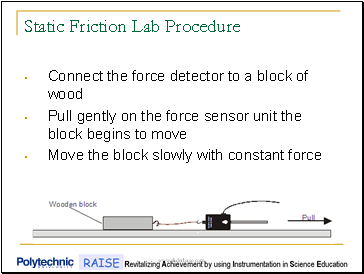Static Friction Lab Procedure

Connect the force detector to a block of wood

Pull gently on the force sensor unit the block begins to move

Move the block slowly with constant force

Slide 11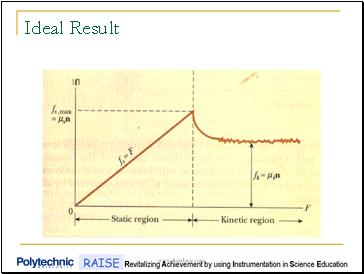Ideal Result

Slide 12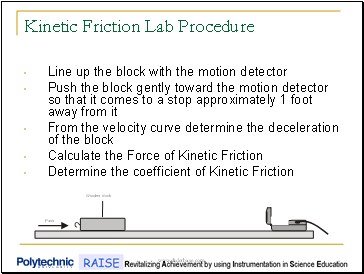Kinetic Friction Lab Procedure

Line up the block with the motion detector

Push the block gently toward the motion detector so that it comes to a stop approximately 1 foot away from it

From the velocity curve determine the deceleration of the block

Calculate the Force of Kinetic Friction

Determine the coefficient of Kinetic Friction

Slide 13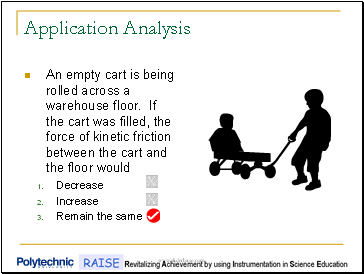Go to page:
1  2